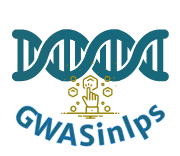GWASinlps performs Bayesian non-local prior based iterative variable selection for data from genome-Wide association studies (GWAS), or other high-dimensional data with continuous, binary or survival outcomes (see References below).

## Installation

### Install from CRAN

install.packages("GWASinlps")

### Install from GitHub

# install.packages("devtools")
devtools::install_github("nilotpalsanyal/GWASinlps")

## The main function:

GWASinlps() is the main function which accepts continuous or binary data (such as phenotype data) and a matrix with the independent variable values (SNP genotypes). The function also needs as input values for scaling parameter of the selected non-local prior and the tuning paramters. These should be fixed based on exploratory study and/or subject-specific heuristics. For example, in GWAS analysis, as the GWAS effect sizes are generally very small (typical effect size of a SNP is around 0.05% of the total phenotypic variance for quantitative traits), the scaling parameter can be chosen such that the non-local prior allows at least 1% chance of a standardized effect size being 0.05 or less in absolute value. Such estimates of the scaling parameter for the MOM and iMOM priors are 0.022 and 0.008, respectively.

Here is a simple illistration of the use the GWASinlps() function for both continous and binary phenotypes.

### GWASinlps analysis with continuous data/phenotypes

library(GWASinlps)
#> This is mgcv 1.8-40. For overview type 'help("mgcv-package")'.
#>
#>  Welcome to GWASinlps! Select well.
#>
#>  Website: https://nilotpalsanyal.github.io/GWASinlps/
#>  Bug report: https://github.com/nilotpalsanyal/GWASinlps/issues

# Generate design matrix (genotype matrix)
n = 200   #number of subjects
p = 10000 #number of variables/SNPs
m = 10    #number of true variables/causal SNPs
set.seed(1)
f = runif( p, .1, .2 )  #simulate minor allele frequency
x = matrix(nrow = n, ncol = p)
for(j in 1:p)
x[,j] = rbinom(n, 2, f[j])  #simulate genotypes
colnames(x) = 1:p

# Generate true effect sizes
causal_snps = sample(1:p, m)
beta = rep(0, p)
beta[causal_snps] = rnorm(m, mean = 0, sd = 2 )

# Generate continuous (phenotype) data
y = x %*% beta + rnorm(n, 0, 1)

# GWASinlps analysis
inlps <- GWASinlps(y=y, x=x, family="normal", prior="mom", tau=0.2,
k0=1, m=50, rxx=0.2)
#> =================================
#> Number of selected variables: 9
#> Time taken: 0.04 min
#> =================================

# LASSO analysis
library(glmnet)
fit.cvlasso = cv.glmnet( x, y, alpha = 1 )
l.min = fit.cvlasso $lambda.min # lambda that gives minimum cvm l.1se = fit.cvlasso$lambda.1se  # largest lambda such that error is
# within 1 se of the minimum

lasso_min = which( as.vector( coef( fit.cvlasso, s = l.min ) )[-1] != 0 )
lasso_1se = which( as.vector( coef( fit.cvlasso, s = l.1se ) )[-1] != 0 )

# Compare results
library(kableExtra)

res = matrix(nrow=3,ncol=3)
res[1,] = c(length(inlps$selected), length(intersect(inlps$selected, causal_snps)), length(setdiff(causal_snps, inlps$selected)) ) res[2,] = c(length(lasso_min), length(intersect(lasso_min, causal_snps)), length(setdiff(causal_snps, lasso_min))) res[3,] = c(length(lasso_1se), length(intersect(lasso_1se, causal_snps)), length(setdiff(causal_snps, lasso_1se))) colnames(res) = c("#Selected SNPs","#True positive","#False negative") rownames(res) = c("GWASinlps", "LASSO min", "LASSO 1se") kableExtra::kable(res, format="html", table.attr= "style='width:60%;'", caption=paste("<center>Variable selection from", p, " SNPs with", m, " causal SNPs for continuous phenotypes from", n, " subjects</center>"), escape=FALSE) %>% kableExtra::kable_styling()  Variable selection from 10000 SNPs with 10 causal SNPs for continuous phenotypes from 200 subjects #Selected SNPs #True positive #False negative GWASinlps 9 8 2 LASSO min 190 8 2 LASSO 1se 44 8 2 ### GWASinlps analysis with binary data/phenotypes library(GWASinlps) library(fastglm) #> Loading required package: bigmemory # Generate design matrix (genotype matrix) n = 500 #number of subjects p = 2000 #number of variables/SNPs m = 10 #number of true variables/SNPs set.seed(1) f = runif( p, .1, .2 ) #simulate minor allele frequency x = matrix(nrow = n, ncol = p) for(j in 1:p) x[,j] = rbinom(n, 2, f[j]) #simulate genotypes colnames(x) = 1:p # Generate true effect sizes causal_snps = sample(1:p, m) beta = rep(0, p) beta[causal_snps] = rnorm(m, mean = 0, sd = 2 ) # Generate binary (phenotype) data prob = exp(x %*% beta)/(1 + exp(x %*% beta)) y = sapply(1:n, function(i)rbinom(1,1,prob[i]) ) # GWASinlps analysis mode(x) = "double" #needed for fastglm() function below mmle_xy = apply( x, 2, function(z) coef( fastglm(y=y, x=cbind(1,matrix(z)), family = binomial(link = "logit")) ) ) #pre-compute MMLEs of betas as it takes time inlps_rigorous <- GWASinlps(y=y, x=x, family="binomial", method="rigorous", mmle_xy=mmle_xy, prior="mom", tau=0.2, k0=1, m=50, rxx=0.2) #> ================================= #> Number of selected variables: 4 #> Time taken: 0.33 min #> ================================= inlps_quick <- GWASinlps(y=y, x=x, family="binomial", method="quick", mmle_xy=mmle_xy, prior="mom", tau=0.2, k0=1, m=50, rxx=0.2) #> ================================= #> Number of selected variables: 8 #> Time taken: 0 min #> ================================= # Lasso analysis library(glmnet) fit.cvlasso = cv.glmnet( x, y, family = "binomial", alpha = 1 ) l.min = fit.cvlasso$lambda.min # lambda that gives minimum cvm
l.1se = fit.cvlasso $lambda.1se # largest lambda such that error is # within 1 se of the minimum lasso_min = which( as.vector( coef( fit.cvlasso, s = l.min ) )[-1] != 0 ) lasso_1se = which( as.vector( coef( fit.cvlasso, s = l.1se ) )[-1] != 0 ) # Compare results library(kableExtra) res = matrix(nrow=4,ncol=3) res[1,] = c(length(inlps_rigorous$selected), length(intersect(inlps_rigorous$selected, causal_snps)), length(setdiff(causal_snps, inlps_rigorous$selected)) )
res[2,] = c(length(inlps_quick$selected), length(intersect(inlps_quick$selected, causal_snps)), length(setdiff(causal_snps, inlps_quick\$selected)) )
res[3,] = c(length(lasso_min), length(intersect(lasso_min, causal_snps)), length(setdiff(causal_snps, lasso_min)))
res[4,] = c(length(lasso_1se), length(intersect(lasso_1se, causal_snps)), length(setdiff(causal_snps, lasso_1se)))
colnames(res) = c("#Selected SNPs","#True positive","#False negative")
rownames(res) = c("GWASinlps rigorous", "GWASinlps quick", "LASSO min", "LASSO 1se")

kableExtra::kable(res, format="html",
table.attr= "style='width:60%;'",
caption=paste("<center>Variable selection from", p, " SNPs with", m, " causal SNPs for binary phenotypes from", n, " subjects</center>"),
escape=FALSE) %>%
kableExtra::kable_styling() 
Variable selection from 2000 SNPs with 10 causal SNPs for binary phenotypes from 500 subjects
#Selected SNPs #True positive #False negative
GWASinlps rigorous 4 4 6
GWASinlps quick 8 4 6
LASSO min 20 5 5
LASSO 1se 6 4 6

Nilotpal Sanyal, Min-Tzu Lo, Karolina Kauppi, Srdjan Djurovic, Ole A. Andreassen, Valen E. Johnson, and Chi-Hua Chen. “GWASinlps: non-local prior based iterative SNP selection tool for genome-wide association studies.” Bioinformatics 35, no. 1 (2019): 1-11. https://doi.org/10.1093/bioinformatics/bty472

Nilotpal Sanyal. “Iterative variable selection for high-dimensional data with binary outcomes.” arXiv preprint arXiv:2211.03190 (2022). https://arxiv.org/pdf/2211.03190.pdf